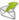Tuesday, October 6, 2015

Making Sense Of An Air Conditioner's EER / COP Efficiency Ratings

If you have ever checked the appliance label on the side of your air conditioner's external unit, you may have noticed the EER and COP ratings. So what are they and how do you use these ratings?

An air conditioner's cooling efficiency is measured by the Energy Efficiency Ratio EER. The EER is the ratio of the cooling capacity output per hour [measured in British thermal units BTU] compared to the input power in watts.

The air conditioner's heating efficiency is measured by the Coefficient Of Performance COP. Both the EER and the COPratings define the output capacity of the appliance, when multiplied by the input power in watts. The higher the EER / COP ratings are, means better appliance efficiency.

When the input power is not stated on the appliance's label, you can work this out by dividing the cooling output capacity figure by the EER and the heating output capacity figure by the COP.

To work out the maximum cost of the appliance, simply multiply this resulting figure by your electricity cost per kWh in cents [example 25 cents].

Cooling example:
• Input power rating 1.84 kW / Output rating 6.0 kW / EER 3.26
• Cost per hour is 1.84 kW X .25 cents = 46 cents
• Input power rating 6.0 / EER 3.26 = 1.84 kW

Heating example:
• Input power rating 2.05 kW / Output rating 7.0 kW / COP 3.41
• Cost per hour 2.05 kW X .25 cents = 51 cents
• Input power rating 7.0 / COP 3.41 = 2.05 kW

The cost per hour in the above example is going to be the maximum cost per hour, as this is not taking account any thermostat operation.

A known rule of thumb calculation used in the world of AC, is to use 70% of this input power rating when the system is reaching the required temperature / thermostat level. Once this level is achieved then the figure is reduced to 40% of the input power rating.

If you are looking for an AC Calculator or want to see example costings then visit this link AIR CONDITIONER COST CALCULATOR.

This blog post brought to you by Aussie Home Energy, a major player in the HOME ELECTRICITY SAVING SERVICES industry in Australia.
Powered byEMF Web Form